### 4. The thermal Sunyaev-Zel'dovich effect

The results in Section 3 indicate that passage of radiation through an electron population with significant energy content will produce a distortion of the radiation's spectrum. In the present section the question of the effect of thermal electrons on the CMBR is addressed in terms of the three likely sites for such a distortion to occur:

 1 the atmospheres of clusters of galaxies 2 the ionized content of the Universe as a whole, and 3 ionized gas close to us.

### 4.1. The Sunyaev-Zel'dovich effect from clusters of galaxies

By far the commonest references to the Sunyaev-Zel'dovich effect in the literature are to the effect that the atmosphere of a cluster of galaxies has on the CMBR. Cluster atmospheres are usually detected through their X-ray emission, as in the example shown in Fig. 2, although the existence of such gas can also be inferred from its effects on radio source morphologies (e.g., Burns & Balonek 1982) - `disturbed' lobe shapes and head-tail sources being typical indicators of the presence of cluster gas.

If a cluster atmosphere contains gas with electron concentration ne (r), then the scattering optical depth, Comptonization parameter, and X-ray spectral surface brightness along a particular line of sight are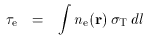(61)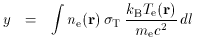(62)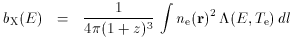(63)

where z is the redshift of the cluster, andis the spectral emissivity of the gas at observed X-ray energy E or into some bandpass centered on energy E (including both line and continuum processes). The factor of 4in the expression for bX arises from the assumption that this emissivity is isotropic, while the (1 + z)3 factor takes account of the cosmological transformations of spectral surface brightness and energy.

By far the most detailed information on the structures of cluster atmospheres is obtained from X-ray astronomy satellites, such as ROSAT and ASCA. Even though these satellites also provide some information about the spectrum of bX (and hence an emission-weighted measure of the average gas temperature along the line of sight) there is no unique inversion of (63) to ne (r) and Te (r). Thus it is not possible to predict accurately the distribution of y on the sky, and hence the shape of the Sunyaev-Zel'dovich effect (which we will, in the current section, take to be close to the shape of y, although Sec. 3.3 indicates that an accurate prediction of the Sunyaev-Zel'dovich effect requires a more complicated calculation which includes both the electron scattering optical depth and the gas temperature).

In many cases it is then convenient to introduce a parameterized model for the properties of the scattering gas in the cluster, and to fit the the values of these parameters to the X-ray data. The integral (62) can then be performed to predict the appearance of the cluster in the Sunyaev-Zel'dovich effect. A form that is convenient, simple, and popular is the isothermalmodel, where it is assumed that the electron temperature Te is constant and that the electron number density follows the spherical distribution(64)

(Cavaliere & Fusco-Femiano 1976, 1978: the so-called `isothermal beta model'). This has been much used to fit the X-ray structures of clusters of galaxies and individual galaxies (see the review of Sarazin 1988). Under these assumptions the cluster will produce circularly-symmetrical patterns of scattering optical depth, Comptonization parameter and X-ray emission, with(65)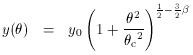(66)(67)

where the central values are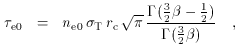(68)(69)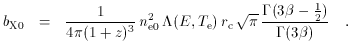(70)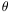is the angle between the center of the cluster and the direction of interest andc = rc / DA is the angular core radius of the cluster as deduced from the X-ray data. DA is the angular diameter distance of the cluster, given in terms of redshift, deceleration parameter q0 , and Hubble constant by(71)

if the cosmological constant is taken to be zero (as it is throughout this review).

A useful variation on this model was introduced by Hughes et al.(1988) on the basis of observations of the Coma cluster. Here the divergence in gas mass which arises for typical values ofthat fit X-ray images is eliminated by truncating the electron density distribution. A model structure of similar form describes the decrease of gas temperature at large radius. The density and temperature functions used are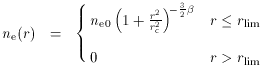(72)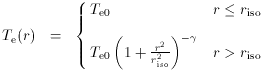(73)

where rlim is the limiting gas radius, riso is the isothermal radius, andis some index. Not all choices of these parameters are physically reasonable, but the forms above provide an adequate description of at least some cluster structures. Further modifications of models (72 - 73) are required in cases where the cluster displays a cooling flow (Fabian et al. 1984), but this will be important only in Sec. 11.1 in the present review.

For cluster CL 0016+16 shown in Fig. 2, the redshift of 0.5455 implies an angular diameter distance DA = 760 h100-1 Mpc (for q0 = 0.5). The X-ray emission mapped with the ROSAT PSPC best matches a circular distribution of form (67) with structural parameters= 0.73 ± 0.02 andc = 0.69 ± 0.04 arcmin, so that rc = (150 ± 10)h100-1 kpc. The corresponding cluster central X-ray brightness, b0 = 0.047 ± 0.002 counts s-1 arcmin-2 (Hughes & Birkinshaw 1998). X-ray spectroscopy of the cluster using data from the GIS on the ASCA satellite and the ROSAT PSPC led to a gas temperature kBTe = 7.6 ± 0.6 keV and a metal abundance in the cluster that is only 0.07 solar. The X-ray spectrum is absorbed by a line-of-sight column with equivalent neutral hydrogen column density NH = (5.6 ± 0.4) x 1020 cm-2. These spectral parameters are consistent with the results obtained by Yamashita (1994) using the ASCA data alone. (5)

Using the known response of the ROSAT PSPC and the spectral parameters of the X-ray emission found from the ASCA data, the emissivity of the intracluster gas in CL 0016+16 ise0 = (2.70 ± 0.06) x 10-13 counts s-1 cm-5. The central electron number density can then be found from (70) to be about 1.2 x 10-2 h1001/2 electrons cm-3. The corresponding central optical depth through the cluster ise0 = 0.01 h100-1/2, which corresponds to a central Comptonization parameter y0 = 1.5 x 10-4h100-1/2. At such a small optical depth, the Sunyaev-Zel'dovich effect through the cluster should be well described by (51), so that the brightness change through the cluster center should beT0 = -2y0Trad-0.82 h100-1/2 mK at low frequency.

More complicated models of the cluster density and temperature can be handled either analytically, or by numerical integrations. For example, Fig. 2 clearly shows a non-circular structure for CL 0016+16: a better representation of the structure of the atmosphere may then be to replace (64) by an ellipsoidal model, with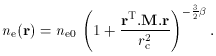(74)

where the matrix M encodes the orientation and relative sizes of the semi-major axes of the cluster. If CL 0016+16 is assumed to be intrinsically oblate, with its symmetry axis in the plane of the sky (at position angle-40°) and with structural parameters that match the X-ray image, then the intrinsic axial ratio is 1.17 ± 0.03, with best-fitting values forand the major axis core radius of 0.751 ± 0.025 and 0.763 ± 0.045 arcmin (Hughes & Birkinshaw 1998). The central X-ray surface brightness is almost unchanged, reflecting the good degree of resolution of the cluster structure effected by the ROSAT PSPC. With these structural parameters, the predicted Sunyaev-Zel'dovich effect map of the cluster is as shown in Fig. 10. The predicted central Sunyaev-Zel'dovich effect is nowT0-0.84h100-1/2 mK at low frequency, very little changed from the prediction of the circular model.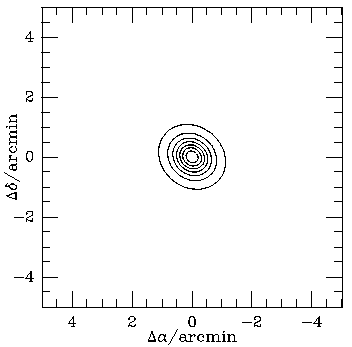Figure 10. A model for CL 0016+16 assuming that the cluster is oblate with the symmetry axis in the plane of the sky, and with the structural parameters fixed by fits to the ROSAT PSPC image (Fig. 2). Left: the model X-ray surface brightness. Right: the model Sunyaev-Zel'dovich effect. The contours in both plots are spaced at intervals of 12.5 per cent of the peak effect - note that the Sunyaev-Zel'dovich effect shows a much greater angular extent than the X-ray emission (compare the angular dependences in equations 66 and 67. The central Sunyaev-Zel'dovich effect predicted on the basis of the X-ray data is -0.84h100-1/2 mK.

Whether (64) or (74) is used to describe the density structure of a cluster, it is important to be aware that these representations are not directly tied to physical descriptions of the gas physics and mass distribution in real clusters, but simply choices of convenience. In principle a comparison of the Sunyaev-Zel'dovich effect map and X-ray image of a cluster could be used to derive interesting information about the structure of the gas, particularly when combined with other information on cluster structure, such as weak lensing maps of the cluster mass distribution, velocity dispersion measurements, and data on the locations of cluster galaxies. Even based on the X-ray and Sunyaev-Zel'dovich effect data alone there are several possibilities for finding out more about cluster structures.

 1 A comparison of the Sunyaev-Zel'dovich and X-ray images might be used to determine the intrinsic three-dimensional shape of the cluster. However, the leverage that the data have on the three-dimensional projection is poor. Changing the model for CL 0016+16 from oblate to prolate only results in a change of about 9 per cent in the central predicted Sunyaev-Zel'dovich effect. Thus this is unlikely to be a useful tool, at least for simple X-ray structures. 2 Since the X-ray emission depends on some average of ne2 along the line of sight, while the Sunyaev-Zel'dovich effect depends on an average of ne, the shape of the Sunyaev-Zel'dovich effect image that is predicted is sensitive to variations of the clumping factor Cn = < ne2 > / < ne > 2 on the different lines of sight through the cluster if clumping occurs at constant gas temperature. The amplitude of the Sunyaev-Zel'dovich effect, indeed, scales as sqrt (Cn). Thus it might be possible to measure the sub-beam scale clumping in the cluster gas. However, if the clumping occurs with a compensating temperature change then the effect may be reduced. For example, if the X-ray emissivity is proportional to Te-1/2 and the clumping is adiabatic, then changes in the X-ray emissivity are matched by equal changes in the Comptonization parameter and no difference will be seen in the Sunyaev-Zel'dovich effect image predicted based on the X-ray data. 3 Probably the most useful astrophysical result that can be extracted from the comparison is information on thermal structure in the intracluster gas. The X-ray and Sunyaev-Zel'dovich effect images depend on Te in different ways, with the X-ray image from a particular satellite being a complicated function of temperature, while the Sunyaev-Zel'dovich effect image is close to being an image of the electron pressure. A comparison of the two images therefore gives information about thermal structure - particularly the thermal structure of the outer part of the cluster, which has a greater fractional contribution to the Sunyaev-Zel'dovich effect than to the X-ray emission (since the X-ray emission depends on ne2 while the Sunyaev-Zel'dovich effect depends on ne). However, it is likely that this information will be more easily gained using spatially-resolved spectroscopy on the next generation of X-ray satellites. 4 Finally, as has been emphasized by Myers et al. (1997), the Sunyaev-Zel'dovich effect is a direct measure of the projected mass of gas in the cluster on the line of sight if the temperature structure of the cluster is simple. This implies that the baryonic surface mass density in the cluster can be measured directly, and compared with other measurements of the mass density, for example from gravitational lensing. A discussion of this in relation to the cluster baryon problem appears in Section 10.

However, most of the recent interest in the Sunyaev-Zel'dovich effects of clusters has not been because of their use as diagnostics of the cluster atmospheres, but rather because the effects can be used as cosmological probes. A detailed explanation of the method and its limitations is given in Section 11, but the essence of the method is a comparison of the Sunyaev-Zel'dovich effect predicted from the X-ray data with the measured effect. Since the predicted effect is proportional to h100-1/2 via the dependence on the angular diameter distance (equation 68 with rc = DAc; see also the discussion of CL 0016+16 above), this comparison measures the value of the Hubble constant, and potentially other cosmological parameters.

It should be emphasized that the Sunyaev-Zel'dovich effect has the unusual property of being redshift independent: the effect of a cluster is to cause some fractional change in the brightness of the CMBR, and this fractional change is then seen at all positions on the line of sight through the cluster, at whatever redshift. Thus the central Sunyaev-Zel'dovich effect through a cluster with the properties of CL 0016+16 will have the same value whether the cluster is at redshift 0.55, 0.055, or 5.5. This makes the Sunyaev-Zel'dovich effect exceptionally valuable as a cosmological probe of hot electrons, since it should be detectable at any redshift for which regions with large electron pressures exist.

There have now been a number of detections of the Sunyaev-Zel'dovich effects of clusters, and recent improvements in the sensitivities of interferometers with modest baselines have led to many maps of the effects. A discussion of the detection strategies, and the difficulties involved in comparing the results from different instruments, is given in Section 8.

5 In this section all errors given in Hughes & Birkinshaw (1998) have been converted to symmetrical ± 1errors, for simplicity. Better treatments of the errors are used in more critical calculations, for example in Sec. 11. Back.